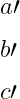# How to write prime(a′) symbol in LaTeX like f′(x)?

Prime symbol is available on your computer keyboard. However, you should also have an idea about the various latex packages and commands.

Symbol Prime symbol
Unicode U+2032
Package Default
Command ′ , \prime, …,etc
Example f\prime(x) → f ′ (x)
\documentclass{article}
\begin{document}
a' b' c'
\end{document}

Output :

a’  b’  c’

Notice the output above, you cannot use the direct prime symbol as a latex command in text mode. Because it will return the single left quotation mark. So, the prime symbol has to be used in math mode.

\documentclass{article}
\begin{document}
a$'$  b$'$  c$'$
$a' b' c'$
\end{document}

Output :

a′  b′  c′
a′  b′  c′


\prime command must be used in math mode. However, it will take the place of inline text. For example

\documentclass{article}
\begin{document}
$a\prime$
$b\prime$
$c\prime$
\end{document}

Output :The above output is not very accurate. Because the prime symbol is always located along the top corner.

So, you can pass in superscript without using a direct prime command. Now you notice the output below.

\documentclass{article}
\begin{document}
$a^{\prime}$
$b^{\prime}$
$c^{\prime}$
\end{document}

Output :

a′
b′
c′


## Double and triple prime symbols in LaTeX

Mathematically, the prime symbol is used in calculus to represent the derivative of a function.

\documentclass{article}
\begin{document}′
$f'(x) \; f^{\prime}(y)$
$f''(x) \; f^{\prime\prime}(y)$
$f'''(x) \; f^{\prime\prime\prime}(y)$
\end{document}

Output :

  f′(x)   f′(y)
f′′(x)   f′′(y)
f′′′(x)   f′′′(y)#### Md Jidan Mondal

LaTeX expert with over 10 years of experience in document preparation and typesetting. Specializes in creating professional documents, reports, and presentations using LaTeX.